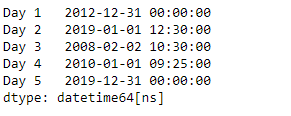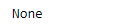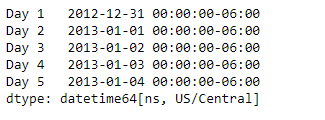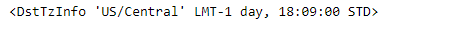Related Articles

# Python | Pandas Series.dt.tz

• Last Updated : 20 Mar, 2019

`Series.dt` can be used to access the values of the series as datetimelike and return several properties. Pandas` Series.dt.tz` attribute return the timezone if any, else it return None.

Syntax: Series.dt.tz

Parameter : None

Returns : timezone

Example #1: Use `Series.dt.tz` attribute to find the timezone of the underlying datetime based data in the given series object.

 `# importing pandas as pd``import` `pandas as pd`` ` `# Creating the Series``sr ``=` `pd.Series([``'2012-12-31'``, ``'2019-1-1 12:30'``, ``'2008-02-2 10:30'``,``               ``'2010-1-1 09:25'``, ``'2019-12-31 00:00'``])`` ` `# Creating the index``idx ``=` `[``'Day 1'``, ``'Day 2'``, ``'Day 3'``, ``'Day 4'``, ``'Day 5'``]`` ` `# set the index``sr.index ``=` `idx`` ` `# Convert the underlying data to datetime ``sr ``=` `pd.to_datetime(sr)`` ` `# Print the series``print``(sr)`

Output :Now we will use `Series.dt.tz` attribute to find the timezone of the datetime data in the given series object.

 `# find the timezone``result ``=` `sr.dt.tz`` ` `# print the result``print``(result)`

Output :As we can see in the output, the `Series.dt.tz` attribute has returned `None` indicating the timezone for the given datetime data is not known.

Example #2 : Use `Series.dt.tz` attribute to find the timezone of the underlying datetime based data in the given series object.

 `# importing pandas as pd``import` `pandas as pd`` ` `# Creating the Series``sr ``=` `pd.Series(pd.date_range(``'2012-12-31 00:00'``, periods ``=` `5``, freq ``=` `'D'``,``                            ``tz ``=` `'US / Central'``))`` ` `# Creating the index``idx ``=` `[``'Day 1'``, ``'Day 2'``, ``'Day 3'``, ``'Day 4'``, ``'Day 5'``]`` ` `# set the index``sr.index ``=` `idx`` ` `# Print the series``print``(sr)`

Output :Now we will use `Series.dt.tz` attribute to find the timezone of the datetime data in the given series object.

 `# find the timezone``result ``=` `sr.dt.tz`` ` `# print the result``print``(result)`

Output :As we can see in the output, the `Series.dt.tz` attribute has successfully returned the timezone of the underlying datetime based data in the given Series object.

Attention geek! Strengthen your foundations with the Python Programming Foundation Course and learn the basics.

To begin with, your interview preparations Enhance your Data Structures concepts with the Python DS Course. And to begin with your Machine Learning Journey, join the Machine Learning – Basic Level Course

My Personal Notes arrow_drop_up